# DATE Function

The DATE function returns the date as a date serial number given a year, month, day.
This function is useful when you need to create dates that will change dynamically.
It is also commonly used to pass dates into other functions rather than using text strings.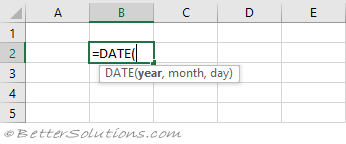### Year, Month, Day

This function takes three arguments and combines them to return a date.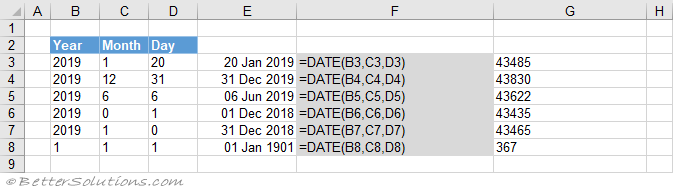### day > 31

You can submit a value that is greater than 31 as the day.
When the day is greater than 31, it is added to the last day of the previous month.
In this example the last day of the previous month is 30 Nov 2019.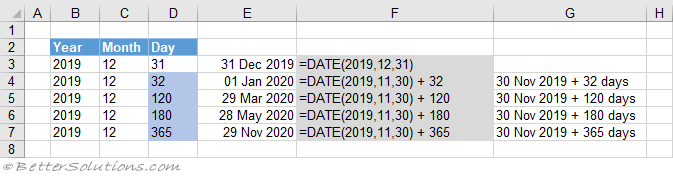### day <= 0

You can submit a value that is less than or equal to zero as the day.
When the day is less than or equal to zero, the number is taken away from the last day of the previous month.
In this example the last day of the previous month is 30 Nov 2019.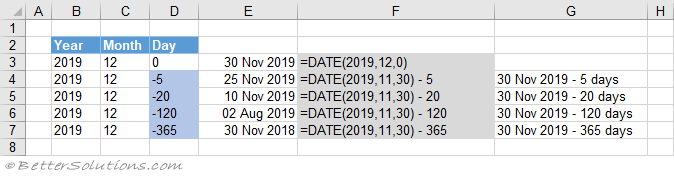### month > 12

You can submit a value that is greater than 12 as the month.
When the month is greater than 12, it is added to the last month of the previous year.
The EDATE is used here to move a certain number of months before or after a date.
In this example the last month of the previous year is Dec 2018.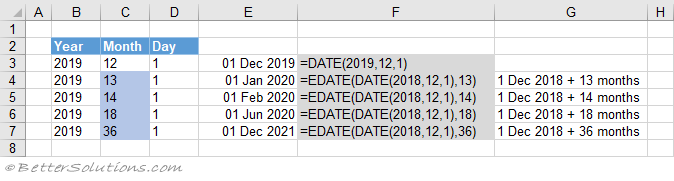### month <= 0

You can submit a value that is less than or equal to zero as the month.
When the month is less than or equal to zero, the number is taken away from the last month of the previous year.
The EDATE is used here to move a certain number of months before or after a date.
In this example the last month of the previous year is Dec 2018.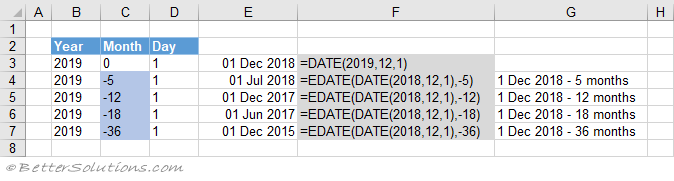### day > 32767

You can submit a value that is greater than 32767 as the day.
When the day is greater than 32767, the number 32767 is used.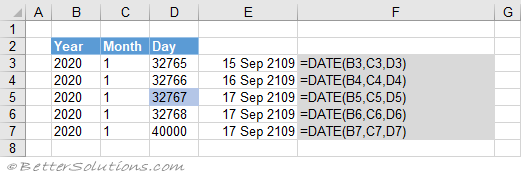### day < -32768

You can submit a value that is less than -32768 as the day.
When the day is less than -32768, the number 32769 (positive) is used.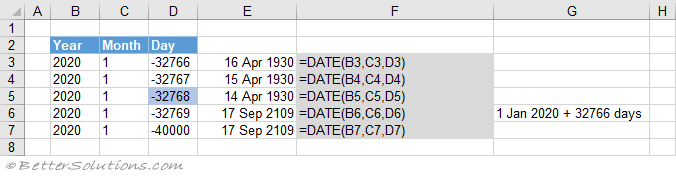Date(1900,1,0)
Date(0,1,1)